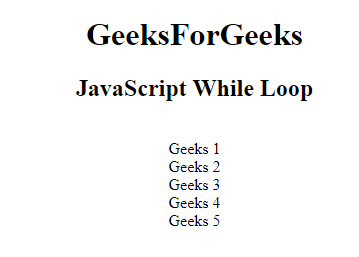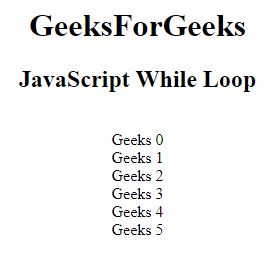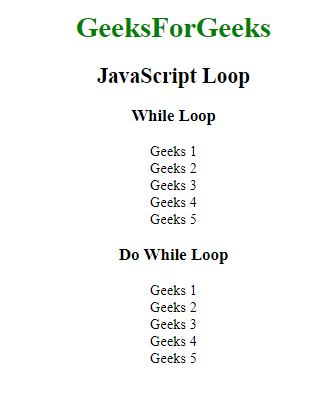# JavaScript | While Loop

While Loop: A while loop is a control flow statement that allows code to be executed repeatedly based on the given Boolean condition. The while loop can be thought of as a repeating if statement.

Syntax:

```while (condition) {
// Statements
}```

Example: This example illustrates the use of while loop.

 ` ` `<``html``> ` ` `  `<``head``>  ` `    ``<``title``> ` `        ``JavaScript While loop ` `    `` ` `  ` ` `  `<``body` `style``=``"text-align:center;"``> ` ` `  `    ``<``div``> ` `        ``<``h1``>GeeksForGeeks ` `         `  `        ``<``h2``>JavaScript While Loop ` `    `` ` `     `  `    ``<``p` `id``=``"GFG"``> ` `     `  `    ``` `    ``<``script``> ` `        ``var print = ""; ` `        ``var val = 1; ` `         `  `        ``while (val < ``6``) { ` `            ``print += "Geeks " + val; ` `            ``print+="
" ` `            ``val+=1; ` `        ``} ` `         `  `        ``document.getElementById("GFG").innerHTML ` `                ``= print; ` `    `` ` ` ` ` `  `                     `

Output:Do-While loop: A do-while loop is a control flow statement that executes a block of code at least once, and then repeatedly executes the block or not depending on a given boolean condition at the end of the block.

Syntax:

```do {
// Statements
}
while (condition);```

Example: This example illustrates the use of do-while loop.

 ` ` `<``html``> ` ` `  `<``head``>  ` `    ``<``title``> ` `        ``JavaScript While loop ` `    `` ` `  ` ` `  `<``body` `style``=``"text-align:center;"``> ` ` `  `    ``<``div``> ` `        ``<``h1``>GeeksForGeeks ` `        ``<``h2``>JavaScript While Loop ` `    `` ` `     `  `    ``<``p` `id``=``"GFG"``> ` `     `  `    ``` `    ``<``script``> ` `        ``var print = "" ` `        ``var val = 0; ` ` `  `        ``do { ` `            ``print += "Geeks " + val; ` `            ``print +="<``br``>"; ` `            ``val+=1; ` `        ``} ` `        ``while (val < ``6``);  ` ` `  `        ``document.getElementById("GFG")``.innerHTML` `                ``= ``print``; ` `    `` ` ` ` ` `  `                     `

Output:Comparison between while and do-while loop: The do-while loop executes the content of the loop once before checking the condition of the while loop. While the while loop will check the condition first before executing the content.

While Loop Do-While Loop
It is an entry condition looping structure. It is an exit condition looping structure.
The number of iterations depends on the condition mentioned in the while block. Irrespective of the condition mentioned in the do-while block, there will a minimum of 1 iteration.
The block control condition is available at the starting point of the loop. The block control condition is available at the end point of the loop.

Example: This example illustrates both while and do-while loop.

 ` ` `<``html``> ` ` `  `<``head``>  ` `    ``<``title``> ` `        ``JavaScript loop ` `    `` ` `  ` ` `  `<``body` `style``=``"text-align:center;"``> ` ` `  `    ``<``div``> ` `        ``<``h1` `style``=``"color:green;"``> ` `            ``GeeksForGeeks ` `        `` ` `         `  `        ``<``h2``>JavaScript Loop ` `    `` ` `     `  `    ``<``h3``>While Loop ` `     `  `    ``<``p` `id``=``"GFG"``> ` `     `  `    ``` `    ``<``script``> ` `        ``var text = ""; ` `        ``var i = 1; ` `        ``while (i < ``6``) { ` `            ``text += "Geeks " + i+"
"; ` `            ``i++; ` `        ``} ` `         `  `        ``document.getElementById("GFG").innerHTML ` `                ``= text; ` `    `` ` `     `  `    ``<``h3``>Do While Loop ` `     `  `    ``<``p` `id``=``"GFG1"``> ` `     `  `    ``` `    ``<``script``> ` `        ``var text = "" ` `        ``var i = 1; ` `     `  `        ``do { ` `            ``text += "Geeks " + i+"<``br``>"; ` `            ``i++; ` `        ``} ` `        ``while (i < ``6``);  ` `     `  `        ``document.getElementById("GFG1")``.innerHTML` `                ``= ``text``; ` `    `` ` ` ` ` `  `                     `

Output:My Personal Notes arrow_drop_upCheck out this Author's contributed articles.

If you like GeeksforGeeks and would like to contribute, you can also write an article using contribute.geeksforgeeks.org or mail your article to contribute@geeksforgeeks.org. See your article appearing on the GeeksforGeeks main page and help other Geeks.

Please Improve this article if you find anything incorrect by clicking on the "Improve Article" button below.

Article Tags :

Be the First to upvote.

Please write to us at contribute@geeksforgeeks.org to report any issue with the above content.